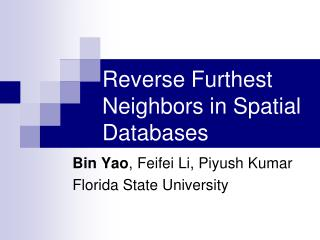# Reverse Furthest Neighbors in Spatial Databases - PowerPoint PPT PresentationDownload PresentationReverse Furthest Neighbors in Spatial Databases

Reverse Furthest Neighbors in Spatial Databases
Download Presentation## Reverse Furthest Neighbors in Spatial Databases

- - - - - - - - - - - - - - - - - - - - - - - - - - - E N D - - - - - - - - - - - - - - - - - - - - - - - - - - -
##### Presentation Transcript

1. Reverse Furthest Neighbors in Spatial Databases Bin Yao, Feifei Li, Piyush Kumar Florida State University

2. A Novel Query Type • Reverse Furthest Neighbors (RFN) Given a point q and a data set P, find the set of points in P that take q as their furthest neighbor • Two versions: • Monochromatic Reverse Furthest Neighbors (MRFN) • Bichromatic Reverse Furthest Neighbors (BRFN)

3. RFN Example : P : Q

4. RFN Application P: a set of customers Q: a set of business competitors offering similar products A distance measure reflecting the rating of customer(p) to competitor(q)’s product. A larger distance indicates a lower preference. For any competitor in Q, an interesting query is to discover the customers that dislike his product the most among all competing products in the market.

5. Furthest Neighbor • Furthest Neighbor: • Given a point p and a set of points Q, p’s furthest neighbor in Q is:

6. MRFN and BRFN • MRFN for q and P: • BRFN for a point q in Q and P are:

7. MRFN: Progressive Furthest Cell Algorithm (first algorithm) Lemma: Any point from the furthest Voronoi cell(fvc) of p takes p as its furthest neighbor among all points in P.

8. Progressive Furthest Cell Algorithm (PFC)PFC(Query q; R-tree T) • Update fvc(q) using points contained by entries in ; • Filter points in using fvc(q); • Initialize two empty vectors and ; priority queue L with T’s root node; fvc(q)=S; • While L is not empty do • Pop the head entry e of L • If e is a point then, update the fvc(q) • If fvc(q) is empty, return; • If e is in fvc(q), then Push e into ; • else • If e fvc(q) is empty then push e to ; • Else for every child u of node e • If u fvc(q) not empty, insert u into L; • Else insert u into ;

9. MRFN: Convex Hull Furthest Cell Algorithm(second algorithm) Lemma: the furthest point for p from Q is always a vertex of the convex hull of Q. In a reverse angle, only vertices of CH have RFN.

10. CHFC(Query q; R-tree T (on P)) // compute only once • Find the convex hull of P; • if then return empty; • else • Compute using ; • Set fvc(q,P*) equal to fvc(q, ); • Execute a range query using fvc(q,P*) on T;

11. Dynamically updating to dataset • PFC: update R-tree • CHFC: • update R-tree (expensive) • Qhull algorithm

12. Dynamically Maintaining CH: insertion

13. Dynamically Maintaining CH: deletion The qhull algorithm

14. Dynamically Maintaining CH Adapt qhull to R-tree

15. BRFN • After resolving all the difficulties for the MRFN problem, solving the BRFN problem becomes almost immediate. • Observations: • all points in P that are contained by fvc(q,Q) will have q as their furthest neighbor. • Only the vertexes of the convex hull have fvc.

16. BRFN algorithm • BRFN(Query q, Q; R-tree T) • Compute the convex hull of Q; • If then return empty; • Else • Compute fvc(q, ); • Execute a range query using fvc(q, ) on T;

17. Disk-Resident Query Group Limitation: query group size may not fit in memory Solution: Approximate convex hull of Q (Dudley’s approximation: the core set idea)

18. Experiment Setup • Dataset: • Real dataset • Synthetic dataset • Measurement • Computation time • Number of IOs • Average of 1000 queries

19. MRFN algorithm CPU computation Number of IOs

20. BRFN algorithms CPU: vary A, Q=1000 IOs: vary A, Q=1000

21. Scalability of various algorithms BRFN number of IOs MRFN number of IOs

22. Conclusion • Introduced a novel query (RFN) for spatial databases. • presented R-tree based algorithms for both version of RFN that feature with excellent pruning capability. • Conducted a comprehensive experimental evaluation.

23. Thank you!Questions?

24. Datasets: San Francisco

25. Datasets: California

26. Datasets: North America

27. Datasets : uncorrelated uniform

28. Datasets : correlated bivariate

29. Datasets : random clusters

30. Motivation • MapQuest, Google Maps, etc. have become essential web services. • Albeit, they provide simple driving directions given a start and an end point. • The same is true for vehicle navigation systems, GPS devices, etc… • It is time to support more advanced services!

31. BRFN algorithms IOs: vary Q,A=3% CPU: vary |Q|,A=3%